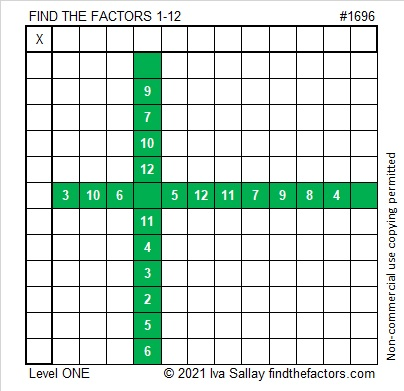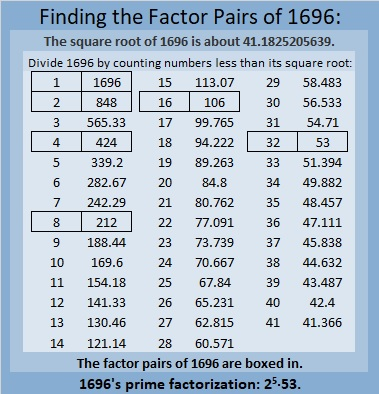# 1696 Inverses

Contents

### Today’s Puzzle:

Joseph Nebus of Nebusresearch recently wrote an extensive post about inverses at my request. Although inverses are often fascinating, advanced topics in mathematics, they can also be quite simple. For example, solving this puzzle will involve using the inverse of multiplication, division, AND it is the simplest division possible this time. Since it is December, I made this puzzle look like a gift for you. Just write the numbers 1 to 12 in both the first column and the top row so that those numbers and the given clues could become a multiplication table.If you want to print the puzzle without color, here it is:### Factors of 1696:

• 1696 is a composite number.
• Prime factorization: 1696 = 2 × 2 × 2 × 2 × 2 × 53, which can be written 1696 = 2⁵ × 53.
• 1696 has at least one exponent greater than 1 in its prime factorization so √1696 can be simplified. Taking the factor pair from the factor pair table below with the largest square number factor, we get √1696 = (√16)(√106) = 4√106.
• The exponents in the prime factorization are 5 and 1. Adding one to each exponent and multiplying we get (5 + 1)(1 + 1) = 6 × 2 = 12. Therefore 1696 has exactly 12 factors.
• The factors of 1696 are outlined with their factor pair partners in the graphic below.### More About the Number 1696:

1696 is the sum of two squares:
36² + 20² = 1696.

1696 is also the hypotenuse of a Pythagorean triple:
896-1440 -1696, which is 32 times (28-45-52).
It can also be calculated from 36² – 20², 2(36)(20), 36² + 20².

1696 is also the difference of two squares in FOUR different ways:
425² – 423² = 1696,
214² – 210² = 1696,
110² – 102² = 1696, and
61² – 45² = 1696.
I found those equations by using the even factor pairs of 1696 and taking the inverse of the fact that a² – b² = (a + b)(a – b).

This site uses Akismet to reduce spam. Learn how your comment data is processed.